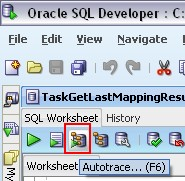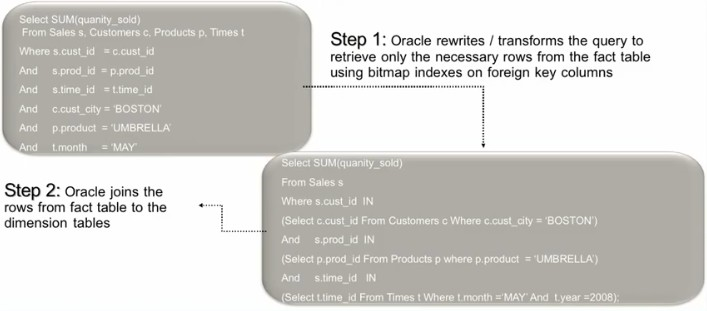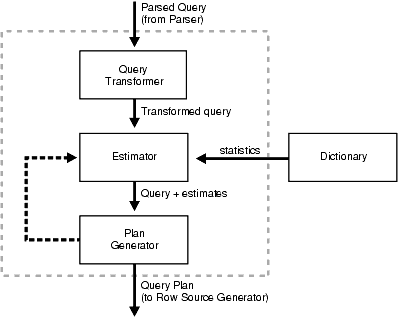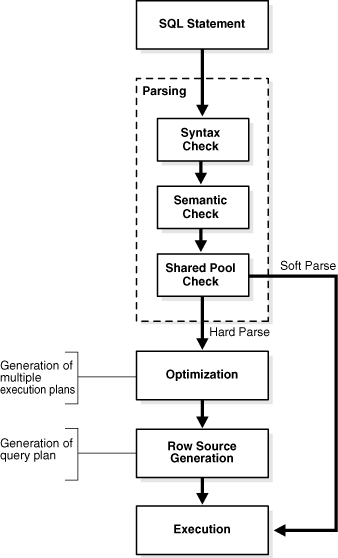# SQL Engine - (Query Plan) Cost

Algorithm - Cost (Performance Metrics) in relational operation.

The cost is an estimated value proportional to the expected resource use needed to execute the statement with a particular execution_plan. The plan estimator calculates the cost of access paths and join orders based on the estimated computer resources, which includes I/O, CPU, and memory.

Serial plans with higher costs take more time to execute than those with smaller costs. When using a parallel plan, however, resource use is not directly related to elapsed time.

The cost represents units of resource used (I/O, CPU, memory).

The query optimizer uses disk I/O, CPU usage, and memory usage as units of work. So, the cost used by the query optimizer represents an estimate of the number of disk I/Os and the amount of CPU and memory used in performing an operation.

The operation can be :

• scanning a table,
• accessing rows from a table by using an index,
• joining two tables together,
• or sorting a row set.

The cost of a query plan is the number of work units that are expected to be incurred when the query is executed and its result produced.

The access path determines the number of units of work required to get data from a base table.

The access path can be :

• a table scan,
• a fast full index scan,
• or an index scan.

During table scan or fast full index scan, multiple blocks are read from the disk in a single I/O operation. Therefore, the cost of a table scan or a fast full index scan depends on the number of blocks to be scanned and the multiblock read count value.

The cost of an index scan depends on the levels in the B-tree, the number of index leaf blocks to be scanned, and the number of rows to be fetched using the rowid in the index keys.

The cost of fetching rows using rowids depends on the index clustering factor.

## Join Cost

The join cost represents the combination of :

• the individual access costs of the two row sets being joined,
• plus the cost of the join operation.

The optimizer estimates costs in the following ways:

• The cost of a nested loops operation is based on the cost of reading each selected row of the outer table and each of its matching rows of the inner table into memory. The optimizer estimates these costs using the statistics in the data dictionary.
• The cost of a sort merge join is based largely on the cost of reading all the sources into memory and sorting them.
• The cost of a hash join is based largely on the cost of building a hash table on one of the input sides to the join and using the rows from the other of the join to probe it.

## Other factors

The optimizer also considers other factors when determining the cost of each operation. For example:

• A smaller sort area size is likely to increase the cost for a sort merge join because sorting takes more CPU time and I/O in a smaller sort area. See “PGA Memory Management” for information on sizing of SQL work areas.
• A larger multiblock read count is likely to decrease the cost for a sort merge join in relation to a nested loop join. If a large number of sequential blocks can be read from disk in a single I/O, then an index on the inner table for the nested loop join is less likely to improve performance over a full table scan. The multiblock read count is specified by the initialization parameter DB_FILE_MULTIBLOCK_READ_COUNT.

Discover MoreCalcite - Query Cost

in calcite. Calcite applies a Cost based optimizer by default that is called the Volcano planner. The cost is provided by the relational expression (relNode). See Cost is represented by org/apache/calcite/plan/RelOptCostRelOptCost...Oracle Database - Autotrace

Autotrace is: a system variable of sql plus can also be found in SQL Developer AUTOTRACE provides you: an execution plan (such as explain plan) and some important regarding its actual execution....Oracle Database - Star Transformation

This article talk the application of the star query in Oracle which involve a star schema. Star transformation executes the query in two phases: retrieves the necessary rows from the fact table (row...Oracle Database - The (index) Clustering Factor Statistics

The (index) Clustering Factor Statistics is a index statistics that indicates the amount of order of the rows in the table based on the values of the index : If the value is near the number of blocks,...Oracle Database - The Query Plan Estimator

The estimator is involved in the query optimizer process. Its main task is to measure the plans that give the plan generator. It generates three different types of measures : These measures...SQL Engine - Optimizer Statistics

In order to make the best execution plan, the optimizer uses statistics on the database objects and the computer system. data dictionary Optimizer statistics are always treated as estimates and can become...SQL Engine - SQL processing (Sql Compiler)

In which way the database processes: DDL statements to create objects, DML to modify data, and queries to retrieve data. Depending on the statement, the database may omit some of these...